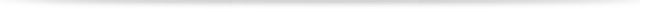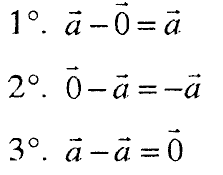# Vectors

#### 1. NOTION OF VECTOR

A segment AB with a starting point A and an end point B is called a directed segment or vector (Fig. 1). The vector AV is denoted AB.

A vector that coincides with the start and end point is called the zero vector and is denoted by 0.

The length of the vector AB is denoted by | AB | and is called the modulus (intensity) of the vector.Fig. 1

The line on which the vector lies determines its direction. Vectors lying on the same line or parallel lines have the same direction. They are said to be collinear vectors.

A vector whose length is a unit is called a unit vector or orth.

For two vectors AB and CD we say that they are equal if they have the same length, the same direction and the same direction.

Two collinear vectors of equal length and opposite directions are called opposite vectors.#### 2. COLLECTION OF VECTORS

For any three points O, A and B in a plane the equation applies

OB = OA + AB

which is called a three-point rule. The vectors OA and AB are called contiguous vectors.

The sum of two (or finite) conjoined vectors is a vector whose beginning is at the beginning of the first and end at the end of the second (last) vector (fig. 2).Fig. 2

Collection properties

1. a + o = a

2. a + (- a) = 0

3. a + b = b + a

(commutative property)

4. (a + b) + c = a + (b + c)

(associative property)#### 3. SUBTRACTION OF VECTORS

The difference of the vectors a and b is the vector x, for which the equation is fulfilled

b – x = a (Fig. Z)Fig.3

Subtraction properties:#### 4. MULTIPLICATION OF VECTOR WITH NUMBER

The product ka = ak of the vector a and the number k ∈ R is a vector b of length | k | ∙ | a |, which for k ≠ 0 and a ≠ 0 is equidistant with a, if k> 0, and opposite to a, if k <o.

Properties of vector multiplication by number:###### – Vector –
Total Page Visits: 236 - Today Page Visits: 2